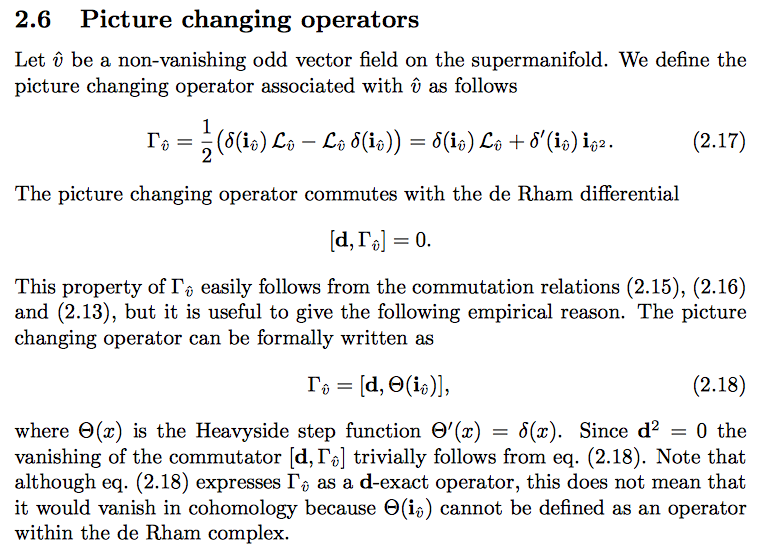# What is the definition of picture changing operation?

What is the definition of picture changing operation? What is a standard reference where it is defined - not just used?

Picture changing operators in supergeometry and superstring theory, Alexander Belopolsky (1997).Although it’s behind an Elsevier pay-wall, there is one paper which explains in cohomological terms the picture-changing operator in the context of string field theory. If I remember correctly it is a kind of connecting homomorphism. The paper in question is “Picture changing operation and BRST cohomology in superstring field theory” by Francisco Narganes-Quijano.

This is a physical description of Picture-Changing Operation:

In the RNS formulation of superstring theory, the worldsheet theory has superconformal gauge invariance. One thus needs to fix the gauge. This means that one can (locally) fix the form of metric and the gravitino field. This will introduce ghost and anti-ghost field into the theory:

• If one fixes diffeomorphism (form of the metric), one gets $(b,c)$ system (they are fermions because they are ghost fields corresponding to a gauge fixing of a Bosonic field);

• If one fixes supersymmetry (form of gravitino), one gets $(\beta,\gamma)$ system (they are bosons because they are ghost fields corresponding to a gauge fixing of a Fermionic field);

The action for the $(\beta,\gamma)$ system has first-order derivative and due to a general properties of such Bosonic systems, the Hamiltonian is unbounded from below which is not a desirable property. To fix this, Friedan, Shenker and Martinec (FSM) introduced an equivalent description of $(\beta,\gamma)$ system in terms of a new set of fields $(\xi,\eta,\phi)$, a process called Bosonization (In this case, it is a Fermionization though, but in the literature it is called Bosonization). The fields $(\beta,\gamma)$ can be written in terms of derivative of $\xi$ and the $\eta$ and $e^{\pm\phi}$ fields. The equivalence of these two description means that OPE of the operators in the two descriptions match. But there is a new feature in this equivalent description : There is a new degree of freedom in $(\xi,\eta,\phi)$ which was somehow hidden in the $(\beta,\gamma)$ description namely the zero mode of $\xi$ field.

This zero mode has crucial importance in string theory :
If this zero mode didn't exist, all Fermionic processes in superstring theory would vanish.

Using ${\bf SL}(2,\mathbb{C})$ invariant vacuum of string theory $\left|0\rangle\right._{{\bf SL}(2,\mathbb{C})}$ (namely the vacuum annihilated by generators of Virasoro algebra $\hat{L}_n$ for $n=0,\pm 1$) and modes of $\beta$ and $\gamma$ fields, one can construct states with arbitrary low energy (due to conformal dimensions of these fields). This means that:

$\left|0\rangle\right._{{\bf SL}(2,\mathbb{C})}$ is not the correct vacuum of the theory.

The correct vacuum of the theory can be written as follows:

$$\left|\boldsymbol{\Omega}\rangle\right.=\hat{c}(0)e^{-\hat{\phi}(0)}\left|0\rangle\right._{{\bf SL}(2,\mathbb{C})}$$

These two states, namely $\left|0\rangle\right._{{\bf SL}(2,\mathbb{C})}$ and $\left|\boldsymbol{\Omega}\rangle\right.$ lives in disjoint Hilbert space (due to the exponential operator), i.e. one can not reach the other by applying finite number of ghost fields modes $\beta_k$ and $\gamma_k$. This means that the states of the original $(\beta,\gamma)$ system contains a condensate of modes of these fields which is called Bose Sea in the language of FSM. They interpreted the charge of the $\phi$ field as the filling level of these Bose sea and it is called Picture Number. On the other hand the vertex operators associated to the the Ramond sector are Spin Fields. The form of spin fields in different picture number is different. There are subtleties on how one can associate picture number to R and NS sectors but all allowed picture numbers gives equivalent description of string theory. If the form of spin field in picture number $\alpha$ is $\mathcal{V}_\alpha$ then one can define the Picture-changing Operation as follows:

$$\mathcal{V}_{\alpha+1}(z)\equiv\{{\hat{Q}}_{\bf BRST},\xi(z)\mathcal{V}_{\alpha}\}$$

One can show that (page 139-140 of Friedan, Shenker and Martinec) two vertex operatos which are related by picture-changing opration give equivalent results for computation of on-shell scattering amplitudes in string theory (section 7 of Friedan, Shenker and Martinec).

For a mathematical description, please check: